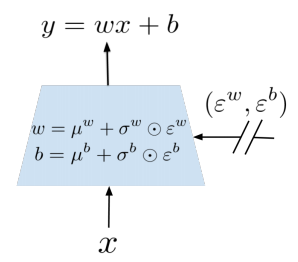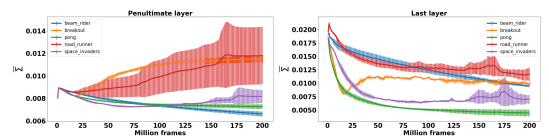# Noisy Network for Exploration

## WHY?

Efficient exploration of agent in reinforcement learning is an important issue. Conventional exploration heuristics includes \epsilon$\epsilon$-greedy for DQN and entropy reward for A3C.

## WHAT?

NoisyNet is a neural network whose parameters are replaced with a parametric function of the noise.\theta =^{def} \mu + \Sigma \odot \epsilon\\ y =^{def} (\mu^w + \sigma^w \odot \epsilon^w)x + \mu^b = \sigma^b \odot \epsilon^b\\$\theta =^{def} \mu + \Sigma \odot \epsilon\\ y =^{def} (\mu^w + \sigma^w \odot \epsilon^w)x + \mu^b = \sigma^b \odot \epsilon^b\\$ There are two options for noise: Independent Gaussian noise and Factorised Gaussian noise. Independent Gaussian noise insert noise per weight(pq + q per layer), but factorised Gaussian insert noise per each input and output(p + q per layer). Since factorised Gaussian requires less random variables generation, it can be used for a single thread agents DQN and independent noise can be used for the distributed A3C. The loss of Noisy Network applied to DQN is as follows.
\mathcal{E}[\mathcal{E}_{(x, a, r, y)\sim D}[r = \gamma Q(y, b^*(y), \epsilon'; \zeta^-) - Q(y, a, \epsilon'; \zeta)]]\\ b^*(y) = argmax_{b\in \mathcal{A}}Q(y, b(y), \epsilon''; \zeta)$\mathcal{E}[\mathcal{E}_{(x, a, r, y)\sim D}[r = \gamma Q(y, b^*(y), \epsilon'; \zeta^-) - Q(y, a, \epsilon'; \zeta)]]\\ b^*(y) = argmax_{b\in \mathcal{A}}Q(y, b(y), \epsilon''; \zeta)$

## So?

NoisyNet-DQN/Dueling/A3C showed overall improved performance over models without noise.The evolution of \sigma$\sigma$ differed significantly by the tasks.

## Critic

I’m quite surprised that this can actually work. More experiments on when the agent choose to explore more would be helpful.

Powered by aiden